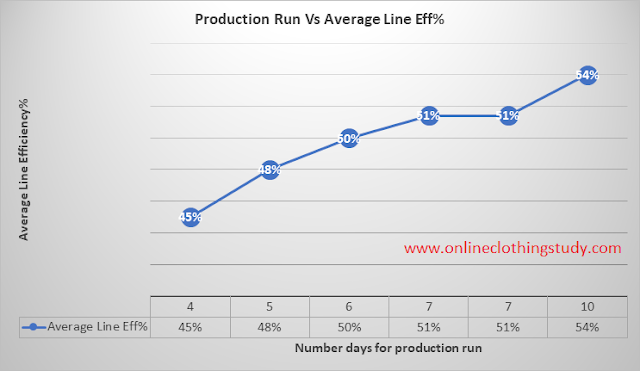# Relation Between Order Quantity and Line Efficiency in Garment Production

It is true that order volume has some impact on the average line efficiency.

It is assumed that if you have a bigger order volume, your average line efficiency will be higher compared to the low volume orders.A couple of OCS readers asked me these questions. In this article, I have explained the relation between order quantity and line efficiency.
• What is the relation between order quantity and line efficiency?
• Application of Quantity factor in garment manufacturing?

Remember: With the garment SAM and order quantity we cannot say what would be the average line efficiency for an order. You need to develop the Quantity Factor by studying your factory’s historical data. Then you can estimate the line efficiency of your lines for an order volume.

Efficiency is measured from actual daily production quantity and manpower involved in the line for making the day's production quantity. It is not calculated or estimated from the order quantity.

To calculate line efficiency, you need to know the total SAM produced by the line and total minutes worked by the line.

Total SAM produced = (Production Qty x SAM)

Total Minutes worked= (Number of employees x Shift time x 60)

If you know the learning curve of your lines (for all the products you make) then you can estimate average line efficiency for orders in advance. Day by day Learning curve efficiency will depend on the product SAM and availability of manpower.

If you do not have a learning curve for your lines, you cannot estimate what would be the average line efficiency for your orders.

I see your orders have different SAM. While making a learning curve you need to consider that also. Secondly, when you have high order volume, you may need to plan to load the style in multiple lines for the same order. This is done to reduce production lead time. The order of 20,000 plus pieces must be loaded in multiple lines.

Here, I will show you how you can develop a relationship between the quantity factor and line efficiency.

If you have daily production data and daily efficiency data of your past orders, you can derive the relationship between order quantity and average line efficiency.

If you do not have such records and data, follow the below steps to develop one.

Let say, learning curve efficiency as following
• Day-1: 30%
• Day-2: 40%
• Day-3: 50%
• Day-4 and onward: 60%
(I am very conservative. For the calculation, I am assuming pick production line efficiency is 60%. In your factory pick efficiency may be 70-80%)

The average line efficiency of an order means - the average of line efficiency from the first day of order loading to the end of the stitching for that order.

To know daily production during the learning curve and after the learning curve, you need to know the manpower and shift time. Let us say 30 operators line working 8 hours shift day.

### Case study:

One line has 500 qty SMV 23, one line has 1500 qty SMV 19, one line has 2000 qty 18, one line has 5000 qty SMV 21, one line has 10000 Qty SMV 28, one line has 15000 qty SMV 25 and one line has 50000 qty SMV 47.

Calculate estimated daily production from Efficiency%, production SAM and available minutes using this formula.

Estimated daily production Quantity= (Available time x Line efficiency)/Garment SAM)

Here, we will consider the learning curve efficiency to calculate the number of days the line completes an order. Then we will calculate average efficiency from the daily efficiency data.

Assume that all lines have the same learning curve and you calculated the number of days needed to complete each order (considering more than one line for high volume orders and orders those need more than 2 weeks to complete production).

### Calculate average line efficiency

So, calculate the average line efficiency for each order.

1. By following the above learning curve first order will be completed in 4 days. Therefore, average line Efficiency = (30+40+50+60)/4= 45
2. Second order will be completed in 5 days. Therefore, average line efficiency for second order = (30+40+50+60+60)/5= 48%
3. Third order will be completed in 6 days. Therefore, average line efficiency= (30+40+50+60+60+60)/6= 50%
4. The 4th order will be completed in 7 days by using two lines.
5. The 5th order will be completed by 7 days by using 3 sewing lines. Therefore, average line efficiency= (30+40+50+60+60+60+60)/7= 51%
6. The 6th order will be completed in 10 days when the order is loaded in 5 lines. Therefore, average line efficiency= (30+40+50+60+60+60+60+60+60+60)/10= 54%Following this method calculate the average of line efficiency for your all orders. In the above table, you can see the daily average line efficiency of the whole order increases when the order volume is increasing. This happens due to the length of the production run.

For this kind of data analysis, real-time production tracking software is very useful.

### Conclusion

We can conclude that when a product run becomes longer, the average line efficiency increases. And the production depends on the order volume and the number of the line used for loading the order.

This case study can be also used to prove that there is the quantity factor (order volume) has a positive impact on the garment manufacturing business.

You can use the above-mentioned steps for developing a relationship between order volume and projected line efficiency.

Related post: Calculate how many days the factory needs to complete order when you have learning curve efficiency data.